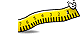Unit Conversion Software Web Widgets Loan Calculator Currency Rates Country Flags Unit Converter
 Faq HelpContact

## Bohr Radius, unit of measure

In the Bohr model of the structure of an atom, put forward by Niels Bohr in 1913, electrons orbit a central nucleus. The model says that the electrons orbit only at certain distances from the nucleus, depending on their energy. In the simplest atom, that of hydrogen, a single electron orbits, and the smallest possible orbit for the electron, that with the lowest energy, is most likely to be found at a distance from the nucleus called the Bohr radius. According to 2002 CODATA, the Bohr radius has a value of 5.291772108(18)*10^-11 m (i.e., approximately 53 pm or 0.53 angstroms). While the Bohr model does not correctly describe an atom, the Bohr radius keeps its physical meaning as a characteristic size of the electron cloud in a full quantum-mechanical description. Thus the Bohr radius is often used as a unit in atomic physics, see atomic units. Note that the definition of Bohr radius does not include the effect of reduced mass, and so it is not precisely equal to the orbital radius of the electron in a hydrogen atom in the more physical model where reduced mass is included. This is done for convenience: the Bohr radius as defined above appears in equations relating to atoms other than hydrogen, where the reduced mass correction is different. If the definition of Bohr radius included the reduced mass of hydrogen, it would be necessary to include a more complex adjustment in equations relating to other atoms.
To perform conversions between Bohr radius and other Distance and Length units please try our Distance and Length Unit ConverterDownload Distance and Length Unit ConverterCopyright © 1998-2009 UnitConversion.org Privacy & Terms | About | Faq | Help | Contact | Link to Us